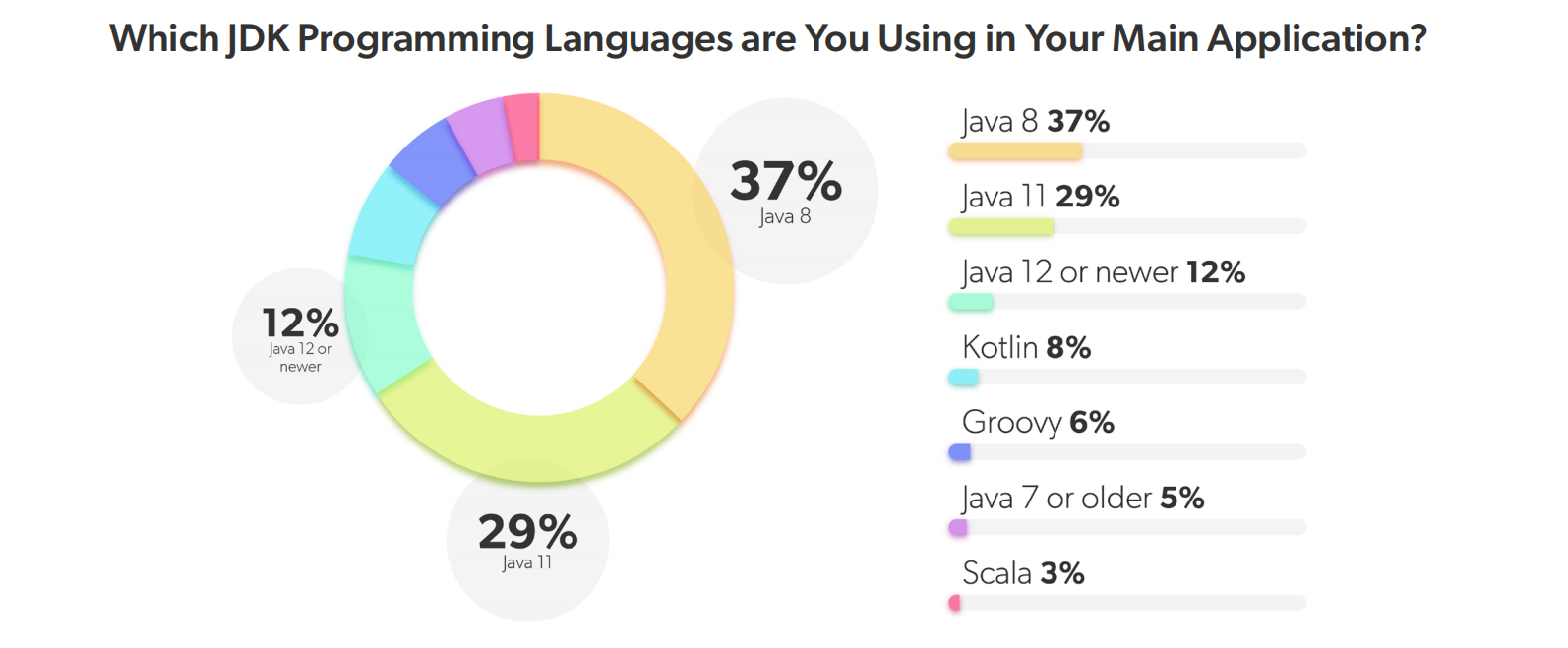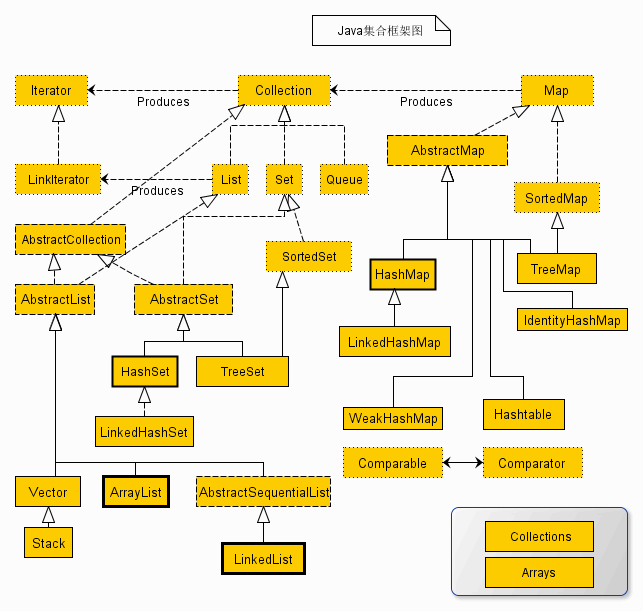Java 编程语言发展迅速，从 Java 9 开始，Java 采取了小步迭代的发布方式，以每 6 个月发布一个版本的速度在持续更新，目前最新的版本已经升到 19 了Java 8 由 Oracle 公司于 2014 年 3 月 18 日发布，在这个版本中新增了大量的特性，首次引入了 Lambda 表达式和方法引用，开启了 Java 语言函数式编程的大门，其中新增的 Stream API（`java.util.stream`）特性更是将函数式编程发挥到了淋漓尽致的地步。

## Stream API 概述

``````List<String> words = List.of("A", "B", "C");
for (String word: words) {
System.out.println(word.toLowerCase());
}``````

``````List<String> words = List.of("A", "B", "C");
Iterator<String> iterator = words.iterator();
while (iterator.hasNext()) {
System.out.println(iterator.next().toLowerCase());
}``````

``````List<String> words = List.of("A", "B", "C");
words.stream().forEach(word -> System.out.println(word.toLowerCase()));``````

``````List<String> names = students.stream()
.filter(s -> s.getScore() >= 60)
.sorted((x, y) -> x.getScore() - y.getScore())
.map(Student::getName)
.collect(Collectors.toList());``````

## Stream API 使用

### 流的创建

JDK 中提供了很多途径来创建一个流，这一节总结一些常用的创建流的方法。流有一个很重要的特性：不会对数据源进行修改，所以我们可以对同一个数据源创建多个流。

#### 创建一个空流

``Stream<String> streamEmpty = Stream.empty();``

``````public Stream<String> streamOf(List<String> list) {
return list == null || list.isEmpty() ? Stream.empty() : list.stream();
}``````

#### 从集合类创建流

JDK 中自带了大量的集合类，比如 `List``Set``Queue` 以及它们的子类，这些类都继承自 `Collection` 接口：``````List<String> collection = Arrays.asList("a", "b", "c");
Stream<String> streamOfCollection = collection.stream();``````

#### 从数组创建流

``````String[] array = new String[]{"a", "b", "c"};
Stream<String> streamOfArray = Arrays.stream(array);``````

``Stream<String> streamOfArray2 = Stream.of(array);``

``Stream<String> streamOfArray3 = Stream.of("a", "b", "c");``

#### 使用 `Stream.builder()` 手工创建流

``````Stream<String> streamOfBuilder = Stream.<String>builder()
.build();``````

``````Stream.Builder<String> builder = Stream.<String>builder();
Stream<String> streamOfBuilder2 = builder.build();``````

#### 使用 `Stream.generate()` 生成流

`Stream.generate()` 方法也可以用于手工创建流，这个方法需要提供一个 `Supplier<T>` 的实现，生成的是一个无限流，一般通过 `limit` 来限定数量：

``Stream<String> streamOfGenerate = Stream.generate(() -> "hello").limit(3);``

``````AtomicInteger num = new AtomicInteger(0);
Stream<Integer> streamOfGenerate2 = Stream.generate(() -> num.incrementAndGet()).limit(3);``````

#### 使用 `Stream.iterate()` 生成流

``Stream<Integer> streamOfIterate = Stream.iterate(1, n -> n + 1).limit(3);``

`iterate()` 函数第一个参数为流的第一个元素，后续的元素通过第二个参数中的 `UnaryOperator<T>` 来迭代生成。

#### 生成基础类型的流

``IntStream intStream = IntStream.range(1, 4);``

``IntStream intStream2 = IntStream.rangeClosed(1, 3);``

``IntStream intStream = new Random().ints(3);``

``````LongStream longStream = new Random().longs(3);
DoubleStream doubleStream = new Random().doubles(3);``````

#### 使用 `String.chars()` 生成字符流

`String` 类提供了一个 `chars()` 方法，用于从字符串生成字符流，正如上面所说，JDK 只提供了 `IntStream``LongStream``DoubleStream` 三种基础类型流，并没有 `CharStream` 一说，所以返回值使用了 `IntStream`

``IntStream charStream = "abc".chars();``

#### 使用 `Pattern.splitAsStream()` 生成字符串流

``Stream<String> stringStream = Pattern.compile(", ").splitAsStream("a, b, c");``

#### 从文件生成字符串流

``````try (Stream<String> stringStream = Files.lines(Paths.get(filePath + "test.txt"));) {
stringStream.forEach(System.out::println);
}``````

### 中间操作

``````Stream<Student> students = Stream.of(
Student.builder().name("张三").gender("男").age(27).number(3L).interests("画画、篮球").build(),
Student.builder().name("李四").gender("男").age(29).number(2L).interests("篮球、足球").build(),
Student.builder().name("王二").gender("女").age(27).number(1L).interests("唱歌、跳舞、画画").build(),
Student.builder().name("麻子").gender("女").age(31).number(4L).interests("篮球、羽毛球").build()
);``````

#### 无状态操作

##### `filter`

`filter` 用于对数据流进行过滤，它接受一个 `Predicate<? super T> predicate` 参数，返回符合该 Predicate 条件的元素：

``students = students.filter(s -> s.getAge() > 30);``
##### `map` / `mapToInt` / `mapToLong` / `mapToDouble`

`map` 接受一个 `Function<? super T, ? extends R> mapper` 类型的参数，对数据流的类型进行转换，从 T 类型转换为 R 类型，比如下面的代码将数据流 `Stream<Student>` 转换为 `Stream<StudentDTO>`

``````Stream<StudentDTO> studentDTOs = students.map(s -> {
return StudentDTO.builder().name(s.getName()).age(s.getAge()).build();
});``````

``LongStream studentAges = students.mapToLong(s -> s.getAge());``

``LongStream studentAges2 = students.mapToLong(Student::getAge);``
##### `flatMap` / `flatMapToInt` / `flatMapToLong` / `flatMapToDouble`

`flatMap` 接受一个 `Function<? super T, ? extends Stream<? extends R>> mapper` 类型的参数，和 `map` 不同的是，他将 T 类型转换为 R 类型的流，而不是转换为 R 类型，然后再将流中所有数据平铺得到最后的结果：

``Stream<String> studentInterests = students.flatMap(s -> Arrays.stream(s.getInterests().split("、")));``

##### `peek`

`peek` 一般用来调试，它接受一个 `Consumer<? super T> action` 参数，可以在流的计算过程中对元素进行处理，无返回结果，比如打印出元素的状态：

``````Stream<String> studentNames = students.filter(s -> s.getAge() > 20)
.peek(System.out::println)
.map(Student::getName)
.peek(System.out::println);``````
##### `unordered`

``````List<Integer> ints = Stream.of(1, 2, 3).unordered()
.map(x -> x*2)
.collect(Collectors.toList());``````

#### 有状态操作

##### `distinct`

`distinct()` 方法用于去除流中的重复元素：

``````Stream<Integer> intStream = Stream.of(1, 2, 3, 2, 4, 3, 1, 2);
intStream = intStream.distinct();``````

`distinct()` 是根据流中每个元素的 `equals()` 方法来去重的，所以如果流中是对象类型，可能需要重写其 `equals()` 方法。

##### `sorted`

`sorted()` 方法根据 自然序（natural order） 对流中的元素进行排序，流中的元素必须实现 `Comparable` 接口：

``````Stream<Integer> intStream = Stream.of(1, 3, 2, 4);
intStream = intStream.sorted();``````

``````students = students.sorted(new Comparator<Student>() {

@Override
public int compare(Student o1, Student o2) {
return o1.getAge().compareTo(o2.getAge());
}

});``````

``students = students.sorted((o1, o2) -> o1.getAge().compareTo(o2.getAge()));``

``students = students.sorted(Comparator.comparing(Student::getAge));``

``````students = students.sorted(
Comparator.comparing(Student::getAge).thenComparing(Student::getNumber)
);``````
##### `skip` / `limit`

`skip``limit` 这两个方法有点类似于 SQL 中的 `LIMIT offset, rows` 语句，用于返回指定的记录条数，最常见的一个用处是用来做分页查询。

``````Stream<Integer> intStream = Stream.of(1, 2, 3, 4, 5, 6, 7, 8, 9, 10);
intStream = intStream.skip(3).limit(3);``````
##### `dropWhile` / `takeWhile`

`dropWhile``takeWhile` 这两个方法的作用也是返回指定的记录条数，只不过条数不是固定的，而是根据某个条件来决定返回哪些元素：

``````Stream<Integer> intStream = Stream.of(1, 2, 3, 4, 5, 6, 7, 8, 9, 10);
intStream = intStream.dropWhile(x -> x <= 3).takeWhile(x -> x <= 6);``````

### 结束操作

#### 短路操作

##### `anyMatch` / `allMatch` / `nonMatch`

`anyMatch` 表示只要有一个元素满足条件即返回 `true`

``boolean hasAgeGreaterThan30 = students.anyMatch(s -> s.getAge() > 30);``

`allMatch` 表示所有元素都满足条件才返回 `true`

``boolean allAgeGreaterThan20 = students.allMatch(s -> s.getAge() > 20);``

`noneMatch` 表示所有元素都不满足条件才返回 `true`

``boolean noAgeGreaterThan40 = students.noneMatch(s -> s.getAge() > 40);``
##### `findFirst` / `findAny`

`findFirst` 用于返回流中第一个元素：

``````// 返回的是 李四
Optional<Student> student = students.filter(s -> s.getAge() > 28).findFirst();``````

`findAny()` 返回的元素是不确定的，如果是串行流，返回的是第一个元素：

``````// 返回的是 李四
Optional<Student> student = students.filter(s -> s.getAge() > 28).findAny();``````

``````// 返回不确定
Optional<Student> student = students.parallel().filter(s -> s.getAge() > 28).findAny();``````

#### 非短路操作

##### `forEach` / `forEachOrdered`

``intStream.forEach(System.out::println);``

``intStream.parallel().forEach(System.out::println);``

``intStream.parallel().forEachOrdered(System.out::println);``
##### `toArray`

`toArray` 方法用于将流转换为一个数组，默认情况下数组类型是 `Object[]`

``Object[] array = students.toArray();``

``Student[] array = students.toArray(Student[]::new);``
##### `reduce`

`reduce` 方法有三种不同的函数形式，第一种也是最简单的：

``Optional<T> reduce(BinaryOperator<T> accumulator);``

``````@FunctionalInterface
public interface BiFunction<T, U, R> {
// ...
}``````

`BinaryOperator` 同样也是两个入参和一个出参的函数，但是它的两个入参的类型和出参的类型是一样的：

``````@FunctionalInterface
public interface BinaryOperator<T> extends BiFunction<T,T,T> {
// ...
}``````

`accumulator` 的意思是累加器，它是一个函数，它有两个参数。它的第一个参数是上次函数执行的返回值（也称为中间结果），第二个参数是流中的元素，函数将两个值按照方法进行处理，得到值赋给下次执行这个函数的参数。第一次执行的时候第一参数的值是流中第一元素，第二个元素是流中第二元素，因为流可能为空，所以这个方法的返回值为 `Optional`

``Optional<Integer> result = students.map(Student::getAge).reduce((x, y) -> x + y);``

``Optional<Integer> result = students.map(Student::getAge).reduce(Integer::sum);``

``Optional<Integer> result = students.map(Student::getAge).reduce((x, y) -> x > y ? x : y);``

``Optional<Integer> result = students.map(Student::getAge).reduce((x, y) -> x < y ? x : y);``

`reduce` 的第二种形式是：

``T reduce(T identity, BinaryOperator<T> accumulator);``

``Integer result = students.map(Student::getAge).reduce(0, Integer::sum);``

`reduce` 的 JDK 源码注释里，有一段伪代码很好地解释了 `reduce` 内部的处理逻辑：

``````U result = identity;
for (T element : this stream)
result = accumulator.apply(result, element)
return result;``````

`reduce` 的第三种形式如下：

``````<U> U reduce(U identity,
BiFunction<U, ? super T, U> accumulator,
BinaryOperator<U> combiner);``````

``Integer result = intStream.parallel().reduce(0, Integer::sum);``

``Integer result = intStream.parallel().reduce(0, Integer::sum, Integer::sum);``

``````Stream<Integer> intStream = Stream.of(1, 3, 2, 4, 2, 4, 2);
Map<Integer, Integer> countMap = intStream.reduce(new HashMap<>(), (x, y) -> {
if (x.containsKey(y)) {
x.put(y, x.get(y) + 1);
} else {
x.put(y, 1);
}
return x;
}, (x, y) -> new HashMap<>());``````

``````Stream<Integer> intStream = Stream.of(1, 3, 2, 4, 2, 4, 2);
List<Integer> distinctMap = intStream.reduce(new ArrayList<>(), (x, y) -> {
if (!x.contains(y)) {
}
return x;
}, (x, y) -> new ArrayList<>());``````

List 转 Map：

``````Map<Long, Student> studentMap = students.reduce(new HashMap<Long, Student>(), (x, y) -> {
x.put(y.getNumber(), y);
return x;
}, (x, y) -> new HashMap<Long, Student>());``````

``````Map<Long, Student> studentMap = students.parallel().reduce(new HashMap<Long, Student>(), (x, y) -> {
x.put(y.getNumber(), y);
return x;
}, (x, y) -> {
for (Map.Entry<Long, Student> entry : y.entrySet()) {
x.put(entry.getKey(), entry.getValue());
}
return x;
});``````
##### `collect`

`collect` 函数正如它的名字一样，可以将流中的元素经过处理并收集起来，得到收集后的结果，这听起来感觉和 `reduce` 函数有点像，而且它的函数定义也和 `reduce` 函数很类似：

``````<R> R collect(Supplier<R> supplier,
BiConsumer<R, ? super T> accumulator,
BiConsumer<R, R> combiner);``````

``````Stream<Integer> intStream = Stream.of(1, 3, 2, 4);
AtomicInteger result = intStream.collect(
() -> new AtomicInteger(),
(a, b) -> {}
);``````

``<R, A> R collect(Collector<? super T, A, R> collector);``

``List<Integer> result = intStream.collect(Collectors.toList());``

``````public static <T>
Collector<T, ?, List<T>> toList() {
return new CollectorImpl<>(
(Supplier<List<T>>) ArrayList::new,
(left, right) -> { left.addAll(right); return left; },
CH_ID);
}``````

``Integer result = intStream.collect(Collectors.summingInt(Integer::valueOf));``

``Optional<Integer> result = intStream.collect(Collectors.maxBy(Integer::compareTo));``

``Map<Integer, Long> result = intStream.collect(Collectors.groupingBy(i -> i, Collectors.counting()));``

``Map<Integer, Integer> result = intStream.collect(Collectors.toMap(i -> i, i -> i, (i, j) -> i));``

List 转 Map：

``Map<Long, Student> result = students.collect(Collectors.toMap(Student::getNumber, Function.identity()));``

``String result = students.map(Student::getName).collect(Collectors.joining("、"));``

``Map<Boolean, List<Student>> result = students.collect(Collectors.partitioningBy(x -> x.getAge() > 30));``

``Map<Integer, List<Student>> result = students.collect(Collectors.groupingBy(Student::getAge));``

`partitioningBy``groupingBy` 函数非常类似，只不过一个将数据分成两组，一个将数据分为多组，它们的第一个参数都是 `Function<? super T, ? extends K> classifier`，又被称为 分类函数（classification function），分组返回的 `Map` 的键就是由它产生的，而对应的 `Map` 的值是该分类的数据列表。很容易想到，既然得到了每个分类的数据列表，我们当然可以继续使用 Stream API 对每个分类的数据进一步处理。所以 `groupingBy` 函数还提供了另一种形式：

``````Collector<T, ?, Map<K, D>> groupingBy(
Function<? super T, ? extends K> classifier,
Collector<? super T, A, D> downstream)``````

``Map<Integer, Long> result = intStream.collect(Collectors.groupingBy(i -> i, Collectors.counting()));``

``````Map<String, List<String>> result = students.collect(Collectors.groupingBy(
Student::getGender, Collectors.mapping(
Student::getName, Collectors.toList())));``````

#### `count`

`count` 比较简单，用于统计流中元素个数：

``long count = students.count();``
##### `max` / `min`

`max``min` 函数用于计算流中的最大元素和最小元素，元素的大小通过比较器 `Comparator<? super T> comparator` 来决定。比如获取年龄最大的学生：

``Optional<Student> maxAgeStudent = students.max(Comparator.comparingInt(Student::getAge));``

``OptionalInt maxAge = students.mapToInt(Student::getAge).max();``
##### `sum` / `average` / `summaryStatistics`

``int sumAge = students.mapToInt(Student::getAge).sum();``

`average` 用于求平均值：

``OptionalDouble averageAge = students.mapToInt(Student::getAge).average();``

`summaryStatistics` 用于一次性获取流中数据的统计信息（包括最大值、最小值、总和、数量、平均值）：

``````IntSummaryStatistics summaryStatistics = students.mapToInt(Student::getAge).summaryStatistics();
System.out.println("Max = " + summaryStatistics.getMax());
System.out.println("Min = " + summaryStatistics.getMin());
System.out.println("Sum = " + summaryStatistics.getSum());
System.out.println("Count = " + summaryStatistics.getCount());
System.out.println("Average = " + summaryStatistics.getAverage());``````

## 更多

### `Collectors` 静态方法一览

• 转换为集合

• `Collector<T, ?, C> toCollection(Supplier<C> collectionFactory)`
• `Collector<T, ?, List<T>> toList()`
• `Collector<T, ?, Set<T>> toSet()`
• 统计计算

• `Collector<T, ?, IntSummaryStatistics> summarizingInt(ToIntFunction<? super T> mapper)`
• `Collector<T, ?, LongSummaryStatistics> summarizingLong(ToLongFunction<? super T> mapper)`
• `Collector<T, ?, DoubleSummaryStatistics> summarizingDouble(ToDoubleFunction<? super T> mapper)`
• `Collector<T, ?, Optional<T>> minBy(Comparator<? super T> comparator)`
• `Collector<T, ?, Optional<T>> maxBy(Comparator<? super T> comparator)`
• `Collector<T, ?, Integer> summingInt(ToIntFunction<? super T> mapper)`
• `Collector<T, ?, Long> summingLong(ToLongFunction<? super T> mapper)`
• `Collector<T, ?, Double> summingDouble(ToDoubleFunction<? super T> mapper)`
• `Collector<T, ?, Double> averagingInt(ToIntFunction<? super T> mapper)`
• `Collector<T, ?, Double> averagingLong(ToLongFunction<? super T> mapper)`
• `Collector<T, ?, Double> averagingDouble(ToDoubleFunction<? super T> mapper)`
• `Collector<T, ?, Long> counting()`
• 字符串拼接

• `Collector<CharSequence, ?, String> joining()`
• `Collector<CharSequence, ?, String> joining(CharSequence delimiter)`
• `Collector<CharSequence, ?, String> joining(CharSequence delimiter, CharSequence prefix, CharSequence suffix)`
• Map & Reduce

• `Collector<T, ?, R> mapping(Function<? super T, ? extends U> mapper, Collector<? super U, A, R> downstream)`
• `Collector<T, ?, T> reducing(T identity, BinaryOperator<T> op)`
• `Collector<T, ?, Optional<T>> reducing(BinaryOperator<T> op)`
• `Collector<T, ?, U> reducing(U identity, Function<? super T, ? extends U> mapper, BinaryOperator<U> op)`
• 分组

• `Collector<T, ?, Map<K, List<T>>> groupingBy(Function<? super T, ? extends K> classifier)`
• `Collector<T, ?, Map<K, D>> groupingBy(Function<? super T, ? extends K> classifier, Collector<? super T, A, D> downstream)`
• `Collector<T, ?, M> groupingBy(Function<? super T, ? extends K> classifier, Supplier<M> mapFactory, Collector<? super T, A, D> downstream)`
• `Collector<T, ?, ConcurrentMap<K, List<T>>> groupingByConcurrent(Function<? super T, ? extends K> classifier)`
• `Collector<T, ?, ConcurrentMap<K, D>> groupingByConcurrent(Function<? super T, ? extends K> classifier, Collector<? super T, A, D> downstream)`
• `Collector<T, ?, M> groupingByConcurrent(Function<? super T, ? extends K> classifier, Supplier<M> mapFactory, Collector<? super T, A, D> downstream)`
• `Collector<T, ?, Map<Boolean, List<T>>> partitioningBy(Predicate<? super T> predicate)`
• `Collector<T, ?, Map<Boolean, D>> partitioningBy(Predicate<? super T> predicate, Collector<? super T, A, D> downstream)`
• List 转 Map

• `Collector<T, ?, Map<K,U>> toMap(Function<? super T, ? extends K> keyMapper, Function<? super T, ? extends U> valueMapper)`
• `Collector<T, ?, Map<K,U>> toMap(Function<? super T, ? extends K> keyMapper, Function<? super T, ? extends U> valueMapper, BinaryOperator<U> mergeFunction)`
• `Collector<T, ?, M> toMap(Function<? super T, ? extends K> keyMapper, Function<? super T, ? extends U> valueMapper, BinaryOperator<U> mergeFunction, Supplier<M> mapSupplier)`
• `Collector<T, ?, ConcurrentMap<K,U>> toConcurrentMap(Function<? super T, ? extends K> keyMapper, Function<? super T, ? extends U> valueMapper)`
• `Collector<T, ?, ConcurrentMap<K,U>> toConcurrentMap(Function<? super T, ? extends K> keyMapper, Function<? super T, ? extends U> valueMapper, BinaryOperator<U> mergeFunction)`
• `Collector<T, ?, M> toConcurrentMap(Function<? super T, ? extends K> keyMapper, Function<? super T, ? extends U> valueMapper, BinaryOperator<U> mergeFunction, Supplier<M> mapSupplier)`
• 其他

• `Collector<T,A,RR> collectingAndThen(Collector<T,A,R> downstream, Function<R,RR> finisher)`